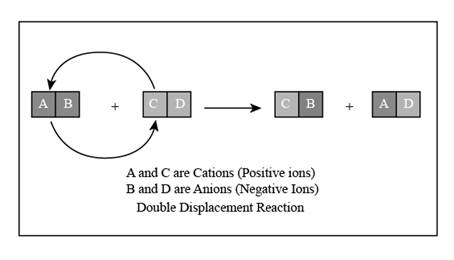Chapter 15, Problem 12ALQ### Introductory Chemistry: A Foundati...

9th Edition
Steven S. Zumdahl + 1 other
ISBN: 9781337399425

#### Solutions

Chapter
Section### Introductory Chemistry: A Foundati...

9th Edition
Steven S. Zumdahl + 1 other
ISBN: 9781337399425
Textbook Problem
87 views

# As with all quantitative problems in chemistry, make sure not to get “lost in the math.” In particular, work on visualizing solutions at a molecular level. For example, consider the following. U have two separate beakers with aqueous solutions, one with 4 units of potassium sulfate and one with 3 “units” of barium nitrate. Draw molecular-level diagrams of both solutions. Draw a molecular-level diagram of the mixture of the two solutions before a reaction has taken place. Draw a molecular-level diagram of the product and solution formed after the reaction has taken place.

Interpretation Introduction

(a)

Interpretation:

The molecular-level diagram of both the solutions is to be drawn.

Concept Introduction:

Compounds formed up of ions are known as ionic compounds. The compound consists of the cation and anion. Cation is the positively charged ion and anion is negatively charged ion. When they are dissolved in a polar solvent like water, the ions of the compounds dissociate in the solvent.

Explanation

Salts on dissolving in water dissociate to give their corresponding cations and anions.

The molecular-level diagram of potassium sulfate is shown in Figure 1

Interpretation Introduction

(b)

Interpretation:

The molecular-level diagram of the solution before the reaction has taken place is to be drawn.

Concept Introduction:

Compounds formed up of ions are known as ionic compounds. The compound consists of the cation and anion. Cation is the positively charged ion and anion is negatively charged ion. When they are dissolved in a polar solvent like water, the ions of the compounds dissociate in the solvent.

Interpretation Introduction

(c)

Interpretation:

The molecular-level diagram of the solution after the reaction has taken place is to be drawn.

Concept Introduction:

The reaction in which two ionic molecules, with their corresponding cations and anions, exchange their counter ions resulting in the formation of two new compounds is known as double displacement reaction. The reaction mechanism can be shown as,Figure 4.

### Still sussing out bartleby?

Check out a sample textbook solution.

See a sample solution

#### The Solution to Your Study Problems

Bartleby provides explanations to thousands of textbook problems written by our experts, many with advanced degrees!

Get Started